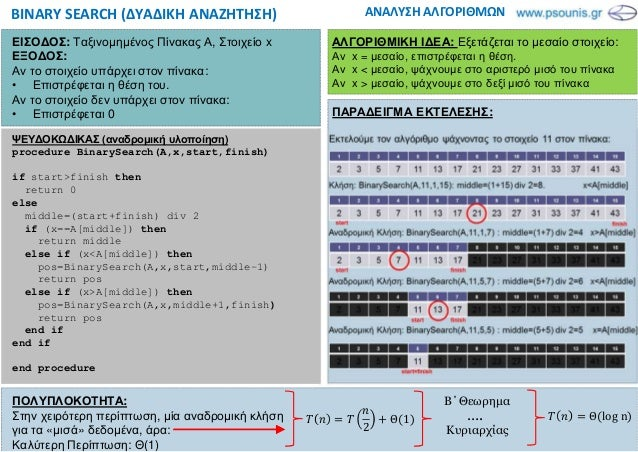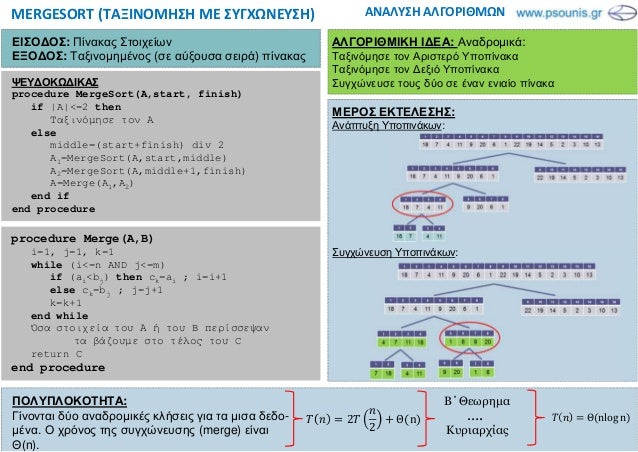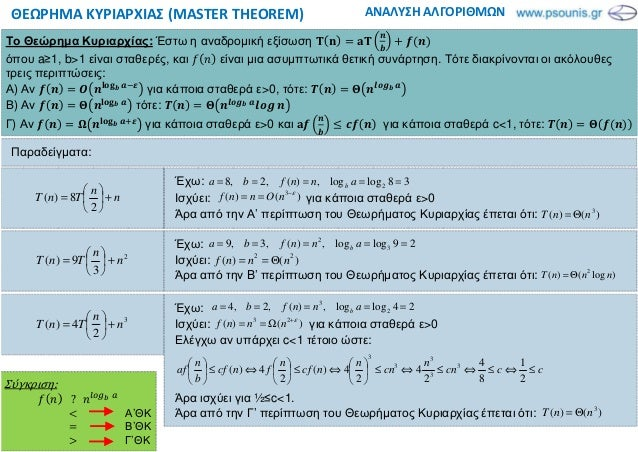Successfully reported this slideshow.Upcoming SlideShare
×

ofUpcoming SlideShare
ΠΛΗ30 ΜΑΘΗΜΑ 1.4 (4in1)
Next

0 Likes

Share

# ΠΛΗ30 ΚΑΡΤΑ ΜΑΘΗΜΑ 1.4

Δυαδική Αναζήτηση (Binary Search)
Ταξινόμηση με Συγχώνευση (Merge Sort)
Θέωρημα Κυριαρχίας (Master Theorem)
Μεθοδος Επανάληψης T(n)=aT(n/b)+f(n)

See all

### Related Audiobooks

#### Free with a 30 day trial from Scribd

See all
• Be the first to like this

### ΠΛΗ30 ΚΑΡΤΑ ΜΑΘΗΜΑ 1.4

1. 1. ΠΟΛΥΠΛΟΚΟΤΗΤΑ: Στην χειρότερη περίπτωση, µία αναδροµική κλήση για τα «µισά» δεδοµένα, άρα: Καλύτερη Περίπτωση: Θ(1) Β΄Θεωρηµα Κυριαρχίας ΠΑΡΑ∆ΕΙΓΜΑ ΕΚΤΕΛΕΣΗΣ: ΑΝΑΛΥΣΗ ΑΛΓΟΡΙΘΜΩΝΒINARY SEARCH (ΔΥΑΔΙΚΗ ΑΝΑΖΗΤΗΣΗ) ΨΕΥ∆ΟΚΩ∆ΙΚΑΣ (αναδροµική υλοποίηση) procedure BinarySearch(A,x,start,finish) if start>finish then return 0 else middle=(start+finish) div 2 if (x==A[middle]) then return middle else if (x<A[middle]) then pos=BinarySearch(A,x,start,middle-1) return pos else if (x>A[middle]) then pos=BinarySearch(A,x,middle+1,finish) return pos end if end if end procedure ΕΙΣΟ∆ΟΣ: Ταξινοµηµένος Πίνακας Α, Στοιχείο x ΕΞΟ∆ΟΣ: Αν το στοιχείο υπάρχει στον πίνακα: • Επιστρέφεται η θέση του. Αν το στοιχείο δεν υπάρχει στον πίνακα: • Επιστρέφεται 0 ΑΛΓΟΡΙΘΜΙΚΗ Ι∆ΕΑ: Εξετάζεται το µεσαίο στοιχείο: Αν x = µεσαίο, επιστρέφεται η θέση. Αν x < µεσαίο, ψάχνουµε στο αριστερό µισό του πίνακα Αν x > µεσαίο, ψάχνουµε στο δεξί µισό του πίνακα 2 Θ 1 Θ log n….
2. 2. ΠΟΛΥΠΛΟΚΟΤΗΤΑ: Γίνονται δύο αναδροµικές κλήσεις για τα µισα δεδο- µένα. Ο χρόνος της συγχώνευσης (merge) είναι Θ(n). Β΄Θεωρηµα Κυριαρχίας ΜΕΡΟΣ ΕΚΤΕΛΕΣΗΣ: Ανάπτυξη Υποπινάκων: Συγχώνευση Υποπινάκων: ΑΝΑΛΥΣΗ ΑΛΓΟΡΙΘΜΩΝMERGESORT (ΤΑΞΙΝΟΜΗΣΗ ΜΕ ΣΥΓΧΩΝΕΥΣΗ) ΨΕΥ∆ΟΚΩ∆ΙΚΑΣ procedure MergeSort(A,start, finish) if |A|<=2 then Ταξινόµησε τον Α else middle=(start+finish) div 2 A1=MergeSort(A,start,middle) A2=MergeSort(A,middle+1,finish) A=Merge(A1,A2) end if end procedure ΕΙΣΟ∆ΟΣ: Πίνακας Στοιχείων ΕΞΟ∆ΟΣ: Ταξινοµηµένος (σε αύξουσα σειρά) πίνακας ΑΛΓΟΡΙΘΜΙΚΗ Ι∆ΕΑ: Αναδροµικά: Ταξινόµησε τον Αριστερό Υποπίνακα Ταξινόµησε τον ∆εξιό Υποπίνακα Συγχώνευσε τους δύο σε έναν ενιαίο πίνακα 2 2 Θ n Θ nlog n…. procedure Merge(A,Β) i=1, j=1, k=1 while (i<=n AND j<=m) if (ai<bj) then ck=ai ; i=i+1 else ck=bj ; j=j+1 k=k+1 end while Όσα στοιχεία του Α ή του B περίσσεψαν τα βάζουµε στο τέλος του C return C end procedure
3. 3. ΑΝΑΛΥΣΗ ΑΛΓΟΡΙΘΜΩΝΘΕΩΡΗΜΑ ΚΥΡΙΑΡΧΙΑΣ (MASTER THEOREM) Το Θεώρηµα Κυριαρχίας: Έστω η αναδροµική εξίσωση όπου a≥1, b>1 είναι σταθερές, και είναι µια ασυµπτωτικά θετική συνάρτηση. Τότε διακρίνονται οι ακόλουθες τρεις περιπτώσεις: Α) Αν για κάποια σταθερά ε>0, τότε: !" Β) Αν τότε: !" !" Γ) Αν # \$ για κάποια σταθερά ε>0 και % & για κάποια σταθερά c<1, τότε: Έχω: Ισχύει: για κάποια σταθερά ε>0 Άρα από την Α’ περίπτωση του Θεωρήµατος Κυριαρχίας έπεται ότι: n n TnT +      = 2 8)( 38loglog,)(,2,8 2 ===== annfba b )()( 3 ε− == nOnnf )()( 3 nnT Θ= Έχω: Ισχύει: Άρα από την B’ περίπτωση του Θεωρήµατος Κυριαρχίας έπεται ότι: 2 3 9)( n n TnT +      = 29loglog,)(,3,9 3 2 ===== annfba b )()( 22 nnnf Θ== )log()( 2 nnnT Θ= Έχω: Ισχύει: για κάποια σταθερά ε>0 Ελέγχω αν υπάρχει c<1 τέτοιο ώστε: Άρα ισχύει για ½≤c<1. Άρα από την Γ’ περίπτωση του Θεωρήµατος Κυριαρχίας έπεται ότι: 3 2 4)( n n TnT +      = 24loglog,)(,2,4 2 3 ===== annfba b )()( 23 ε+ Ω== nnnf cccn n cn n ncf n fncf b n af ≤⇔≤⇔≤⇔≤      ⇔≤      ⇔≤      2 1 8 4 2 4 2 4)( 2 4)( 3 3 3 3 3 )()( 3 nnT Θ= Σύγκριση: ? 1234 5 6 Α’ΘΚ Β’ΘΚ 7 Γ’ΘΚ Παραδείγµατα:
4. 4. ΑΝΑΛΥΣΗ ΑΛΓΟΡΙΘΜΩΝΜΕΘΟΔΟΣ ΕΠΑΝΑΛΗΨΗΣ Παράδειγµα: Να λύσετε την αναδροµή: Τ 9 3 ; < = 7 1 0 1 Λύση: Τ 3 ; < = 3 3 ; <? ; < = = 3= ; <? 3 ; < = = 3= 3 ; <@ ; <? = 3 ; < = = 3A ; <@ 3= ; <? = 3 ; < = = ⋯ 3C ; <D 3C E ; <DFG = ⋯ 3= ; <? = 3 ; < = = Η αναδροµή σταµατά όταν ; <D 1 ⇒ n 4C ⇒ k log< 3KLMN ; ; <OPQN R 3KLMN ; E ; <OPQN RFG = ⋯ 3= ; <? = 3 ; < = = 3KLMN ; 1 3KLMN ; E ; <OPQN RFG = ⋯ 3= ; <? = 3 ; < = = ∑ 3T ; <U =KLMN ; E TVW ∑ 3T ;? <U ? KLMN ; E TVW = ∑ AU <? U KLMN ; E TVW = ∑ AU EXU KLMN ; E TVW = ∑ A EX TKLMN ; E TVW = @ GY OPQN R E @ GY E Θ = 1. Κάνουµε 3 εφαρµογές της αναδροµικής σχέσης (µέχρι να φτάσουµε στη µορφή: Τ n ZA ; [@ ). Χρήσιµο το πρόχειρο 2. Εκτίµηση της σειράς που προκύπτει µετά από k επαναλήψεις (µας καθοδηγεί ο όρος: ; [D ) 3. Υπολογίζουµε πότε σταµατάει η αναδροµή (Θέτουµε ; [D W και λύνουµε ως προς k). Π.χ. αν W 1, τότε k log[ 4. Αντικατάσταση του k στον τύπο του βήµατος 2 5. Υπολογισµός της σειράς που προκύπτει. Χρήσιµος ο τύπος: ∑ T; TVW ]R^G E ] E Η µέθοδος της επανάληψης χρησιµοποιείται για την επίλυση της αναδροµικής σχέσης _ `a , 7 c &, c • Όταν το ζητάει ρητά η εκφώνηση • Όταν αποτυγχάνει το θεώρηµα κυριαρχίας • Όταν δεν θέλουµε απλά µία ασυµπτωτική εκτίµηση Τ 3 ; < = ; < 3 ; <? ; < = ; <? 3 ; <@ ; <? = Πρόχειρο: 0

Total views

8,613

On Slideshare

0

From embeds

0

Number of embeds

7,000

375

Shares

0